Fill Gauge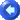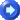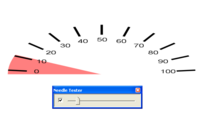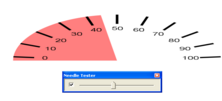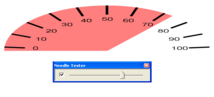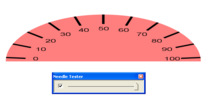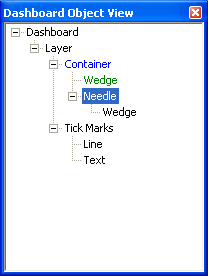This is a simple gauge shown here in one layer.  Set your center points to be the same for your needle, wedge, and tick marks.

 OBJECT PROPERTIES Container Clip to first child box enabled Wedge (child of container) Horizontal Radius=20 Vertical Radius=20 Wedge Angle=180 Wedge Sweep=180 Needle Type=Circular Sweep=180 Range=0 to 100 Wedge (child of needle) Horizontal Radius=20 Vertical Radius=20 Wedge Angle=0 Wedge Sweep=180 Pen Style=Transparent Brush Color=(255, 0, 0) Brush Opacity=128 Brush Style=Solid Tick Marks Type=Circular Tick Count=11 Range=0 to 100 Horizontal Radius=20 Vertical Radius=20 Angle=185 Sweep=170 Line (Default Options) Text (Default Options)